StatLect

Conditional probability mass function

The probability distribution of a discrete random variable can be characterized by its probability mass function (pmf). When the probability distribution of the random variable is updated, in order to consider some information that gives rise to a conditional probability distribution, then such a conditional distribution can be characterized by a conditional probability mass function.Definition

The following is a formal definition.

Definition Letandbe two discrete random variables. The conditional probability mass function ofgivenis a function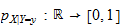such thatfor any, whereis the conditional probability that, given that.

How to compute the conditional pmf

In order to derive the conditional pmf of a discrete variablegiven the realization of another discrete variable, we need to know their joint probability mass function.

Suppose that we are informed that, wheredenotes the value taken by(called the realization of).

How do we take this information into account? By deriving the conditional probability mass function of.

The derivation involves two steps:

1. first, we compute the marginal probability mass function ofby summing the joint probability mass over the support of(i.e., the set of all its possible values, denoted by):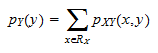2. then, we compute the conditional pmf as follows: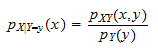An example

Here is an example.

Take two discrete variablesandand consider them jointly as a random vectorSuppose that the support of this vector is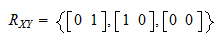and that its joint pmf is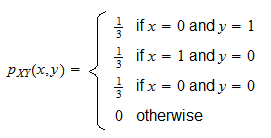Let us compute the conditional pmf ofgiven.

The support ofisThe marginal pmf ofevaluated atis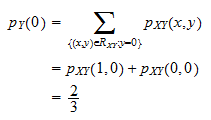The support ofisTherefore, the conditional pmf ofconditional onis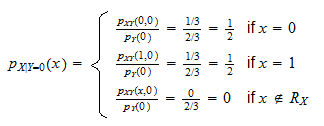How to derive the joint pmf from the conditional and marginal

The previous example showed how the conditional pmf can be derived from the joint pmf. We can easily do the other way around.

If we know the marginal pmfand the conditional, then we can multiply them and obtain the joint distribution: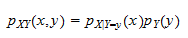More details

You can find more details about the conditional probability mass function in the lecture entitled Conditional probability distributions.